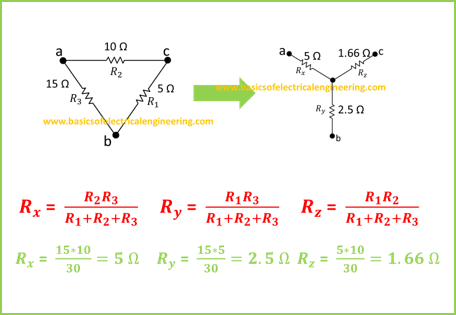# Basics of Electrical Engineering

Learn the basics of Electrical Engineering.

The delta and wye connections are two special arrangments which are often used in electrical circuits. In this post, you'll learn about the conversions between these two circuits.

We previously studied about the series, parallel and series-parallel circuit configurations. The delta and wye conversions are two other configurations which are used in electrical circuits. Most of the times conversion between these two forms is required. In this post, you'll learn about the delta-wye conversion formulas with examples.

Let's solve an example for the learning process.Here five different examples (final solutions) are provided for the sake of practice. Convert the delta to wye for practice and match the answers.
Sr Delta (R1, R2, R3) Wye (Rx, Ry, Rz)
1. 6, 14, 16 6.22, 2.66, 2.33
2. 10, 50, 100 31.25, 6.25, 3.125
3. 18, 36, 42 15.75, 7.875, 6.75
4. 3, 6, 9 3, 1.5, 1
5. 166, 255, 331 112.240, 73.066, 56.289